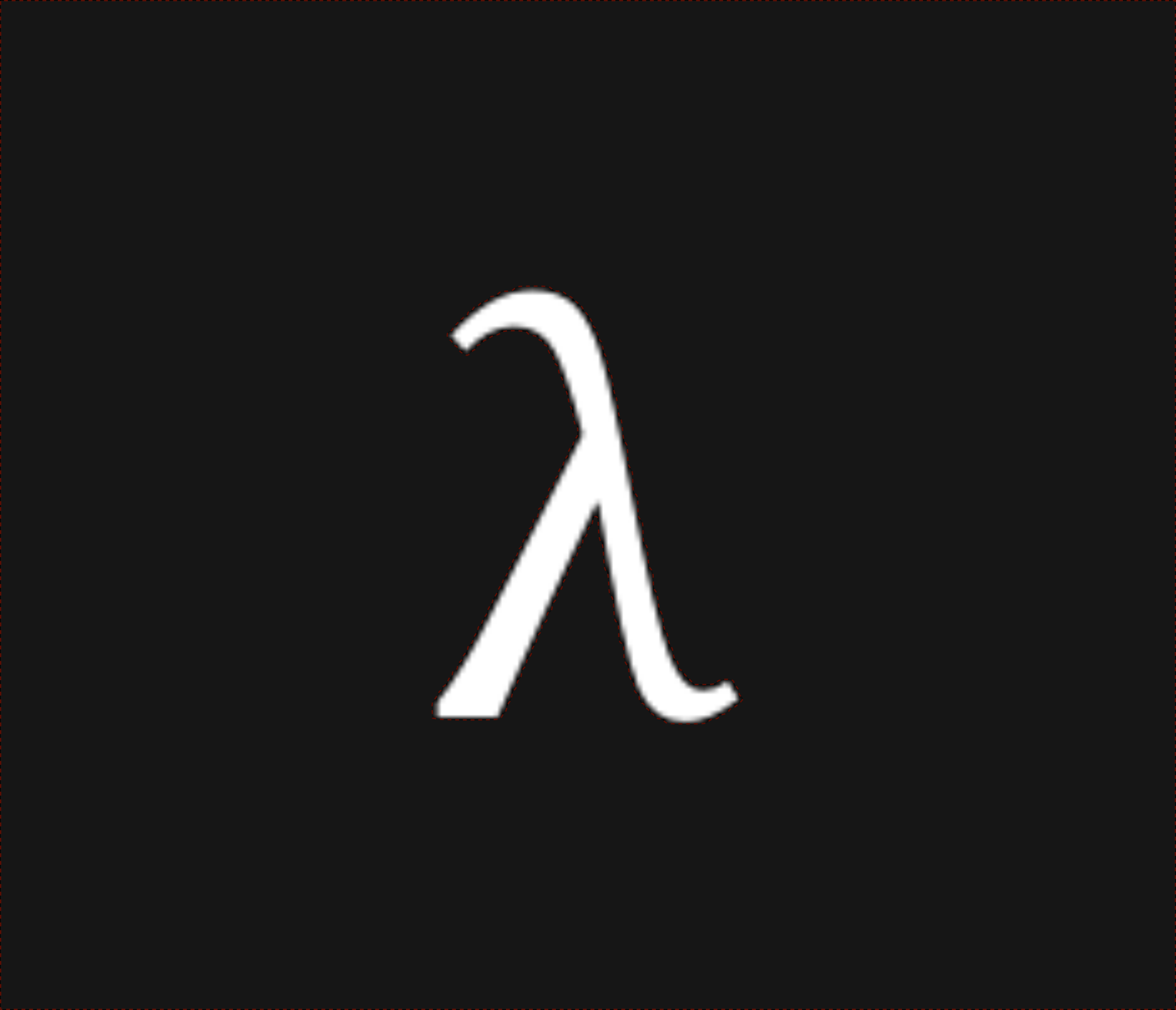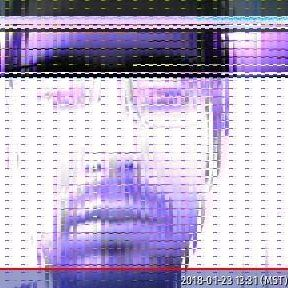nixpulvis

Overview of Mathematics

Mathematics makes use of symbols and names somewhat liberally, allowing for a certain amount of conciseness and expressiveness, but not always disambiguation. Notion can be context sensitive, be careful.

Also, this is not a guide, or a lesson. I’m mostly trying to catalog useful notation and expressions.

1. Logic
1. Symbols
2. Rules
3. Proofs
2. Mathematics
1. Notation
1. Objects
2. Operations & Expressions
3. Conventions
2. Subjects
1. Algebra
2. Calculus
3. Linear Algebra
4. Statistics
5. Combinatorics
6. Set/Group/Category Theory
7. Real Analysis*
3. Programming
4. Graphics and Plotting
5. Abstraction & Application

Logic

Logic is the foundation of all reasoning and thought. Though, not all logics are created equal.

Formal logic denotes a collection of symbols and rules for these symbols. Used carefully, one can start here and build whole models of mathematics and thus anything.

Symbols

Negation: $\neg A$, $!A$

“Not” is written as $\neg$ (or $!$ by programmers). For example, all roads do not lead to Rome. Let’s call this proposition $\neg R$.

Equivalence: $A \equiv B$, $A \leftrightarrow B$

We could say that all roads do in fact lead to Rome, and that would be $\neg \neg R \equiv R$. This could also be written as $\neg \neg R \leftrightarrow R$ (or even $\neg \neg R \sim R$ in Kleene’s “Mathematical Logic”).

Junction: $A \land B$, $A \lor B$

“And” and “Or” are written as $\land$, and $\lor$, respectively. We obviously can’t claim $R \land \neg R$ is true.

Implication: $A \Rightarrow B$, $A \rightarrow B$

(also $A \supset B$ in Kleene’s “Mathematical Logic”)

Entailment: $\vDash A$

We can now formally state in our observer language, $\nvDash R \land \neg R$, for example.

Approximation: $\sim A$, $\approx A$, $O$

Somewhere along the way to Rome I might ask, “How long has it been since we left camp?”, which, unless someone or something was perfectly counting, could only be met with $\sim 12$ hours. It might also be useful to write $t \approx 12$. Looking over at the travelers along side us, we recognize a few from hours ago when we left. We could say our distance ($d_1$) and their distance ($d_2$) are related as $d_1(t) \sim d_2(x)$, or even $O(d_1(t)) = O(d_2(t))$.

Mathematics

Notation

Objects

Numbers are the primary objects of math. You can use numbers to count, for example, $1, 2, 3$, Go! You can use numbers for measures of units, for example, the current temperature $65°F$.

Fractions are for frogs, $\frac{3}{7}$, ribbit.

Operations & Expressions

Math is full of various operations you can write into valid (or invalid) mathematical notation. Values can be computed, like $\pi^3$, or $\sqrt 2$.

Equations

All hail the mighty equal sign, $=$. Simply put, the equal sign says two things are identical, or equal. We call this an equation.

A really obvious equation might be $2 + 2 = 4$, while we can represent something as complex as the concept of a limit as simply as $1 = 0.9999999\dots$ or $1 = 0.\overline{999}$.

Variables

Many equations have letters in them, which can allow a fixed value to change with the variable, for example $x^2$ grows quickly, but $2^x$ even faster. Some variables are used to represent physical, or conceptual properties, for example $a^2 + b^2 = c^2$ describes the edge lengths of a triangle, where $a$ and $b$ and the sides, and $c$ is the hypotenuse.

Vectors and Matrices

Vectors come in a many forms. For example as a $\vec{v}$ or as three (in this case) coefficients along with component unit vectors starting with $\hat{i}, \hat{j}, \hat{k}, \ldots$.

Vectors may also be written in matrix form as $A$ or $B$.

Matrices are just vectors of vectors.

Transpose

Scalar Multiplication

Matrix Multiplication

Exponentiation

Inverse

Limits

A $\lim_{x\to\infty}$ is a way to talk about the convergence of something.

Integration

Integral $\int_{a}^{b} x^2 dx$ inside text looks nice too.

Cases

This is where things can get “fun”.